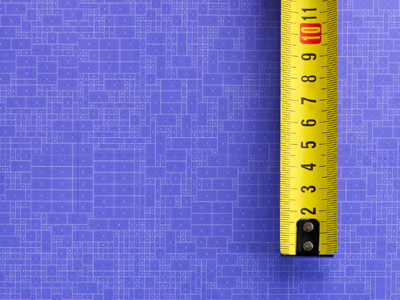There are 10 millimetres in a centimetre.

# Decimal Numbers (Year 5)

In KS2 Maths, kids learn about decimal numbers. In Year Five, they explore the value of digits in decimals, like tenths and hundredths. Plus, they practice adding and subtracting decimals. Rounding decimals up or down becomes a breeze, and they get comfy with sums involving decimal measurements such as metres/cm or £/pence.

Decimal numbers are smaller than one and come after a decimal point. They're like another way of writing fractions. For example, a quarter of pizza as a fraction is 1/4, but as a decimal, it's 0.25. The 2 in 0.25 means 2 tenths, and the 5 is 5 hundredths.

Can you spot the difference between tenths and hundredths? How about rounding a number to two decimal places? Test your decimal number skills by playing this quiz!

1.
What is the figure 6 worth in 0.26?
6 hundredths
6 ones
6 tenths
6 tens
Remember - tenths, hundredths, thousandths
2.
Which is the largest?
0.35
0.05
0.03
0.3
35100 is larger than 30100, 5100 or 3100
3.
What is the next number in the following sequence? 0.24, 0.26, 0.28, 0.3
0.32
0.35
1.3
1.32
The numbers are going up by 0.02, or 2100 every time
4.
How is 3.57 changed to 3.6?
Subtract 0.3
Subtract 0.03
Just like 357 + 3 = 360
3.57 + 0.03 = 3.6
5.
Which is a decimal fraction halfway between 2.1 and 2.2?
2.12
2.13
2.14
2.15
There are ten hundredths in a tenth so half of one tenth is 5 hundredths
6.
How is 0.07 changed to 0.02?
Subtract 0.5
Subtract 0.05
Just like 7 - 5 = 2
0.07 - 0.05 = 0.02
7.
Which is the decimal fraction equivalent to seven and forty two hundredths?
7.042
7.42
0.742
74.2
Forty two hundredths is equivalent to four tenths and two hundredths
8.
What does the digit 2 represent in 5.12?
2 tens
2 tenths
2 ones
2 hundredths
In decimals the first column after the ones is tenths and the second column is hundredths
9.
What is 8.5cm in mm?
8.5mm
85mm
850mm
8,500mm
There are 10mm in 1cm therefore we multiply by 10 to find the answer
10.
Which is the smallest of these numbers?
0.12
1.02
0.02
0.01
1100 is less than 2100, 12100 and 102100